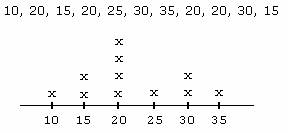# 4 Ways To Calculate Mode In Java | Java Programs

Java Program To Calculate Mode – In this article, we will brief in on the mentioned means to calculate the mode of given data in Java Programming. Following java code has been written in multiple ways for easy understand.

• Using Scanner Class
• Using Static Method
• Using Command Line Arguments
• Using Separate Class

Just like Median, Mode is the value in a given set of probability distributions or populations which is repeated the most number of times.

Mode specifies the maximum occurring value in the set of options given. If there is no value repeating more than the others, then the set has no mode.As you can see in the example given above, this is a list having multiple values.

10, 20, 15, 20, 25, 30, 35, 20, 20, 30, 15.

It is clearly visible that the number 20 is repeated 4 times. Hence, the mode of this list is 20.

## Mode Java Code

Scanner Class in Java is very well known for reading inputs at runtime. For this problem, we require an array to store values and hence, we first take input of length/size of the array (n) followed by which we read the elements in the array (a).

To find the mode, we first require to find the number of times each element of the array is repeated. To store this count, we need another array (b) of same size.

The next step is, we take each element of the array and compare it with all the elements of the array.

If the element is equal to another element in the array then the count is incremented (variable used here is c) and the final value of count (c) is stored in array b for the same index (i) as the element compared.

To avoid redundancy and repetition of counting the same element twice when we find another element equal to the element compared, as soon as count is incremented the another element (a[j]) value is made equal to -1.

And not just that, every time we encounter element -1 the count for that index in array b (b[i]) is automatically equated to 0 as goes to the next element.

Once all the elements in array (a) are compared and all the count values in another array (b) are updated, then the task is to find the index having the largest count. For this, we initially set the index to zero and begin comparing with all the other elements.

If an element it found to be greater than this, then the index is updated to the index of this newly found largest element. This process continues till we reach the end of array.

The index of the largest count is then used to find the element in array (a) positioned at the same index. This element is the mode that we are looking for.

Output

Using Static Method

Static method makes use of the same logic referred above. The difference arises in the writing of code. Unlike the above, here we do not write the entire code in the same main method but instead, split it up.

There will be another static method in the same class as that of main method which will consists of the set of statements used for finding the count of each element in the array and then finding the largest count to find the most repeated number in that array i.e., the mode.

But, to give this method all the inputs is taken care of in the main method using the scanner class.

The scanner class reads the size of array and the elements of array.

This input is this passed to the static method (modeCal) which will be called in the main method.

This executes the set of instructions written within it and returns the output mode which is then displayed by using output statement in the main method.

Output:

### Using Command Line Arguments

In the command line arguments, while giving the command to run the code along with the name of the code we also give the size of array (arg) with a space followed by the data values of the array (arg[i+n]).

These arguments are taken by main method are are stored in respective variables like the size of array here is stored in integer variable ‘n’ and the data values are stored in array (a) of size n. While storing we also convert them into their respective data type using parsing method like

After storing these inputs, we perform the same set of instructions as used above.

x

## Plus Star Pattern Java Program | Patterns

Java program to print plus star pattern program – We have written the below print/draw ...